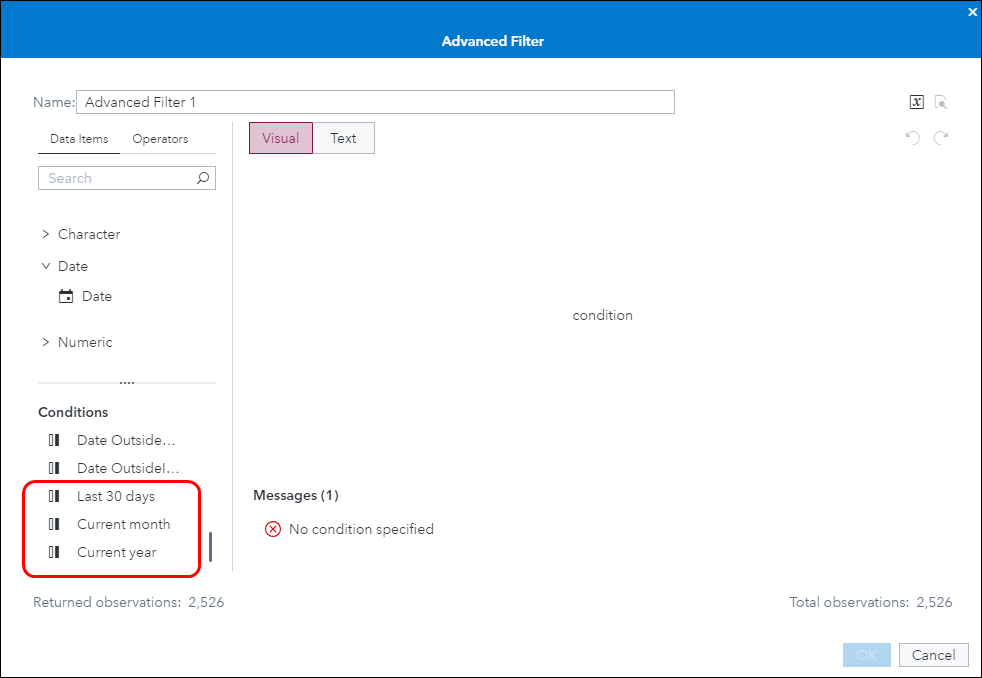## subtract numeric value from month parameter in SAS VA

Dear friends, Looking forward for some help in below under SAS VA.

I have Period drop down with values 'January' , 'February' ....

I am keeping storing this value in Month parameter.

I have another drop down  that can select flag values like 1 , 3 , 6 and 12.

Based on selection of this field, final List table must subtract current period with selected flag value and show the result.

Eg, if I select Period as March, and flag as 3 , list must show Jan , Feb and March data,

If i select Period as March and flag as 1 , list must show only March data.

basically it should show periods between selected period and  selected period - flag.

Kindly let me know if anyone has any idea on this.

Based on Period selection and below filter is giving data from selected period to beginning of period.

Month('Month'p) >= Month('Period'n)

7 REPLIES 7

## subtract numeric value from month parameter

Dear friends, Looking forward for some help in below under SAS VA.

I have Period drop down with values 'January' , 'February' ....

I am keeping storing this value in Month parameter.

I have another drop down  that can select flag values like 1 , 3 , 6 and 12.

Based on selection of this field, final List table must subtract current period with selected flag value and show the result.

Eg, if I select Period as March, and flag as 3 , list must show Jan , Feb and March data,

If i select Period as March and flag as 1 , list must show only March data.

basically it should show periods between selected period and  selected period - flag.

Kindly let me know if anyone has any idea on this.

Based on Period selection and below filter is giving data from selected period to beginning of period.

Month('Month'p) >= Month('Period'n)

## Re: subtract numeric value from month parameter

MODERATOR: Please move this to the SAS Visual Analytics forum.

--
Paige Miller

## Re: subtract numeric value from month parameter

@PaigeMiller wrote:

MODERATOR: Please move this to the SAS Visual Analytics forum.

Done

--------------------------
The hash OUTPUT method will overwrite a SAS data set, but not append. That can be costly. Consider voting for Add a HASH object method which would append a hash object to an existing SAS data set

Would enabling PROC SORT to simultaneously output multiple datasets be useful? Then vote for
Allow PROC SORT to output multiple datasets

--------------------------Sam_SAS
SAS Super FREQ

## Re: subtract numeric value from month parameter in SAS VA

Hello,

I don't think there is an easy way in VA to take a month value like JAN2023 and subtract months from it accurately for an advanced filter. The challenge is when we cross the boundary between years.

SAS date values are stored as the number of days since 01JAN1960, so it would be possible to subtract a given number of days from the selected month, like 30, 90, 180, or 365. That is probably the best fit for what you are trying to do.

Something else you might consider would be the periodic operators for a calculated measure, such as CumulativePeriod(). You could use these with IF/ELSE logic to show the current month, current quarter, current year, etc., selectable from a drop-down or button bar control. Or you could present all three of those as separate aggregated measures in the same object.

Let us know if that helps. Working with dates can be tricky in Visual Analytics, and there have a number of threads on this community board about the subject.

Sam

## Re: subtract numeric value from month parameter in SAS VA

Thanks a lot for your kind response. I am exactly looking for logic to subtract x days from given period in SAS VA. I have now parameterized rolling flages to return 30 ,90 etc.

## Re: subtract numeric value from month parameter in SAS VA

Below formulae is giving error Type mismatch: expected type is Boolean, type of item being applied is numeric.

Period is values like January, February..

TreatAs(_Number_, month('Period'n)) - rolling_month

or

TreatAs(_Number_, month('Period'n)) - 30Sam_SAS
SAS Super FREQ

## Re: subtract numeric value from month parameter in SAS VA

Hello,

Are you putting the expression into the advanced filter editor, or the calculated item editor?

The expression for a filter needs to return a boolean (true/false) so it would need to be something like:

TreatAs(_Number_, month('Period'n)) - rolling_month > 0     (or whatever would actually make sense)

There needs to be a comparison operator like >, <, =, or In.

The Month() operator requires a date value, so your Period parameter would need to be a date parameter. If you apply a month format to the date, it would still display values like 'January'.

In order to subtract days, your Period also will need to be a date. The date value could have a month/year format with values like JAN2023, if that helps.

All in all, this is not an easy thing to do in VA.

In the Advanced Filter window, under Conditions, you will find these:If you select 'Last 30 days' it will generate syntax like this:

'Date'n >= TreatAs(_Date_, ( TreatAs(_Number_, DatePart(Now())) - 30 ))

You can use this as a starting point to build an expression. You can substitute your Period parameter for the "DatePart(Now())"

'Date'n >= TreatAs(_Date_, ( TreatAs(_Number_, 'Period'p) - 30 ))

Building on that, you could code some IF/ELSE logic to handle the rolling_month parameter:

IF 'rolling_month'p = 1

RETURN 'Date'n >= TreatAs(_Date_, ( TreatAs(_Number_, 'Period'p) - 30 ))

ELSE IF 'rolling_month'p = 3

RETURN 'Date'n >= TreatAs(_Date_, ( TreatAs(_Number_, 'Period'p) - 90 ))

{and so on}

This should work, it will just take a little time and trial and error to get it working the way that you want.

Sam

Discussion stats
• 7 replies
• 404 views
• 0 likes
• 4 in conversation